# ARC (Command)

Creates an arc.

Find

To create an arc, you can specify combinations of center, endpoint, start point, radius, angle, chord length, and direction values. Arcs are drawn in a counterclockwise direction by default. Hold down the Ctrl key as you drag to draw in a clockwise direction.

The following prompts are displayed.

### Start point

Draws an arc using three specified points on the arc's circumference. The first point is the start point (1).

Note: If you press ENTER without specifying a point, the endpoint of the last drawn line or arc is used and you are immediately prompted to specify the endpoint of the new arc. This creates an arc tangent to the last drawn line, arc, or polyline.
Second point
Specify the second point (2) is a point on the circumference of the arc.
End point
Specify the final point (3) on the arc.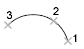You can specify a three-point arc either clockwise or counterclockwise.

### Center

Starts by specifying the center of the circle of which the arc is a part.

Start point
Specify the start point of the arc.
End point

Using the center point (2), draws an arc counterclockwise from the start point (1) to an endpoint that falls on an imaginary ray drawn from the center point through the third point (3).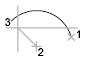The arc does not necessarily pass through this third point, as shown in the illustration.

Angle

Draws an arc counterclockwise from the start point (1) using a center point (2) with a specified included angle. If the angle is negative, a clockwise arc is drawn.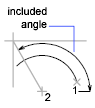Chord length

Draws either a minor or a major arc based on the distance of a straight line between the start point and endpoint.

If the chord length is positive, the minor arc is drawn counterclockwise from the start point. If the chord length is negative, the major arc is drawn counterclockwise.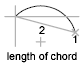### End

Starts by specifying the endpoint of the arc.

Center point

Draws an arc counterclockwise from the start point (1) to an endpoint that falls on an imaginary ray drawn from the center point (3) through the second point specified (2).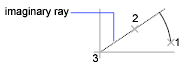Angle

Draws an arc counterclockwise from the start point (1) to an endpoint (2), with a specified included angle. If the angle is negative, a clockwise arc is drawn.

Included angle
Enter an angle in degrees or specify an angle by moving the pointing device counterclockwise.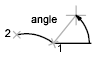Direction

Begins the arc tangent to a specified direction. It creates any arc, major or minor, clockwise or counterclockwise, beginning with the start point (1), and ending at an endpoint (2). The direction is determined from the start point.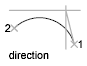Draws the minor arc counterclockwise from the start point (1) to the endpoint (2). If the radius is negative, the major arc is drawn.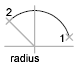### Center

Specifies the center of the circle of which the arc is a part.

Start point
Specify start point of arc.
End point

Draws an arc counterclockwise from the start point (2) to an endpoint that falls on an imaginary ray drawn from the center point (1) through a specified point (3).Angle

Draws an arc counterclockwise from the start point (2) using a center point (1) with a specified included angle. If the angle is negative, a clockwise arc is drawn.Chord length

Draws either a minor or a major arc based on the distance of a straight line between the start point and endpoint.

If the chord length is positive, the minor arc is drawn counterclockwise from the start point. If the chord length is negative, the major arc is drawn counterclockwise.### Tangent to last line, arc, or polyline

Draws an arc tangent to the last line, arc, or polyline drawn when you press ENTER at the first prompt.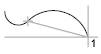End point of arc
Specify a point (1).